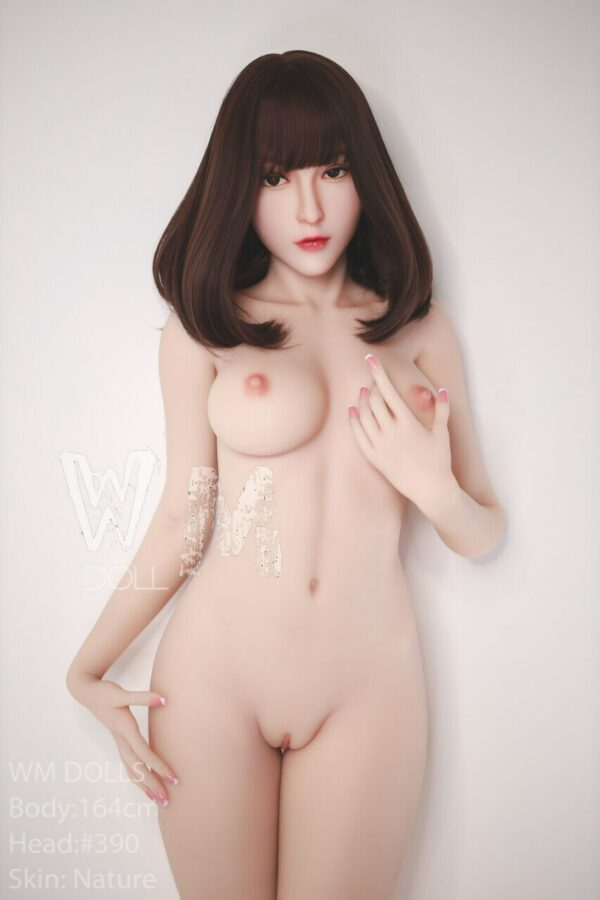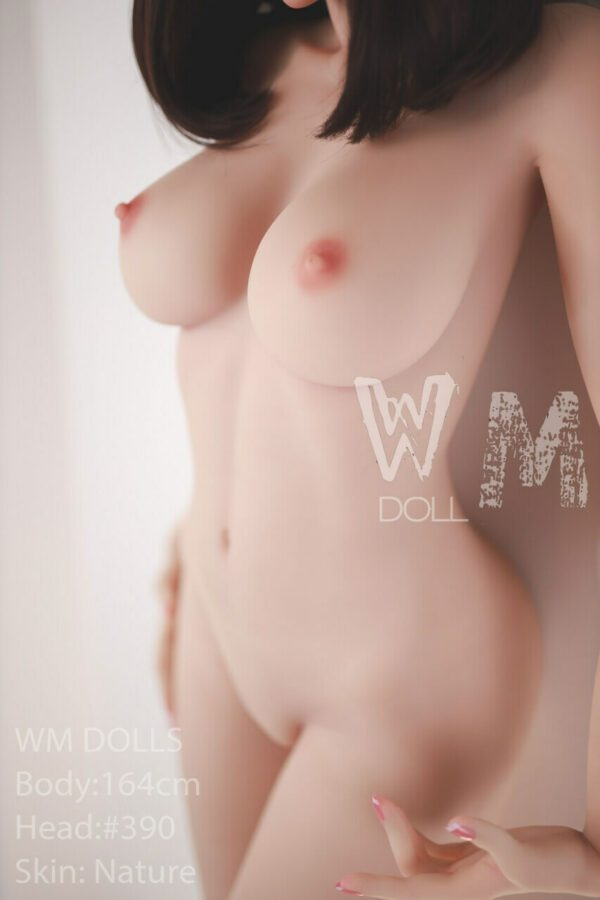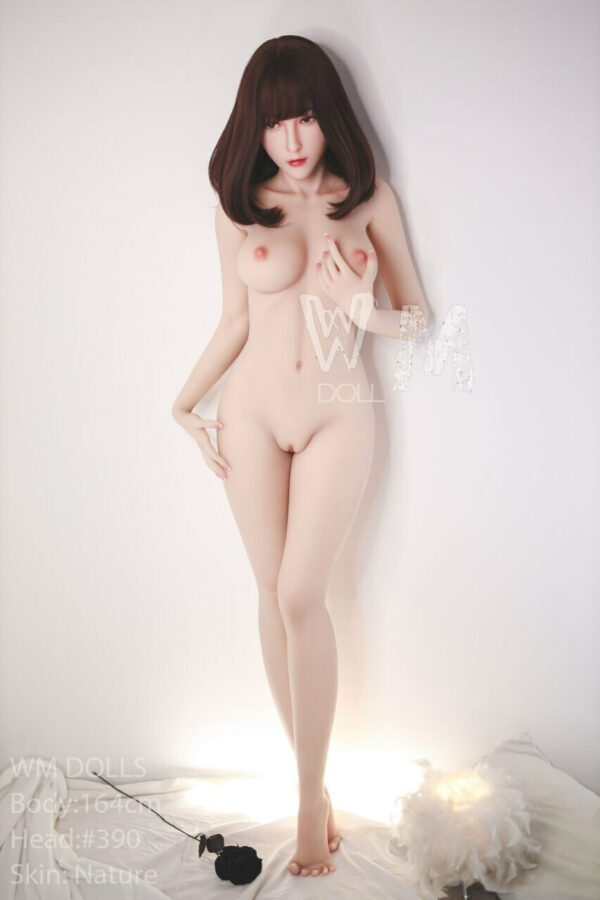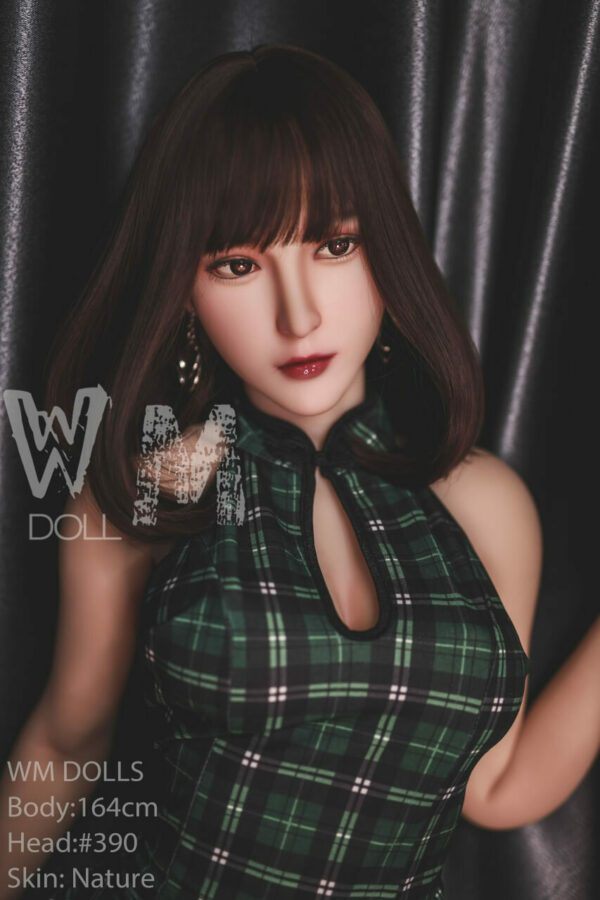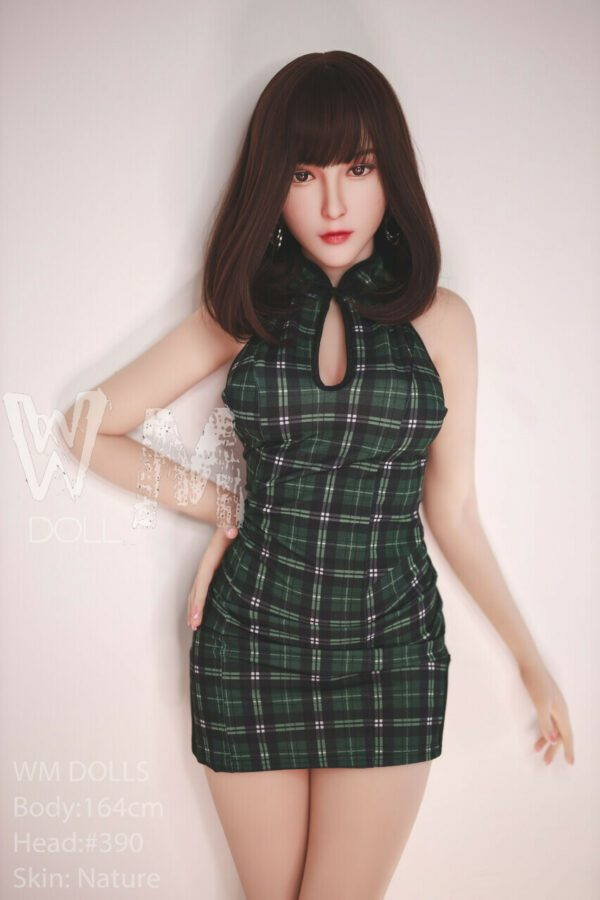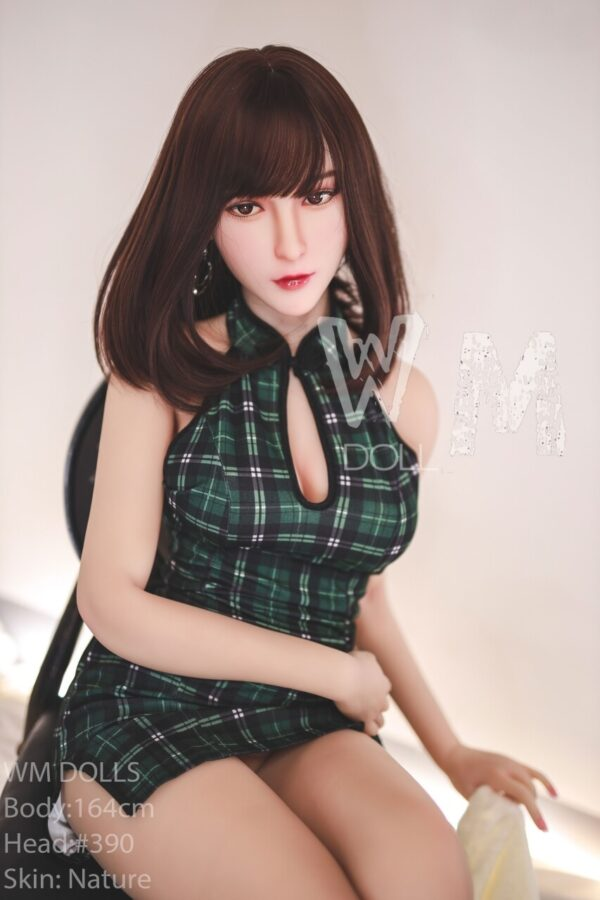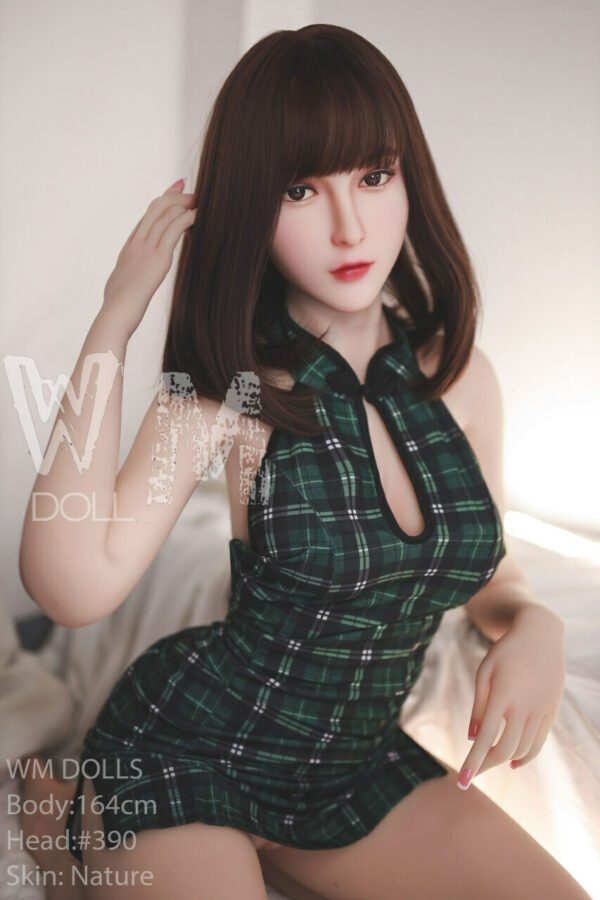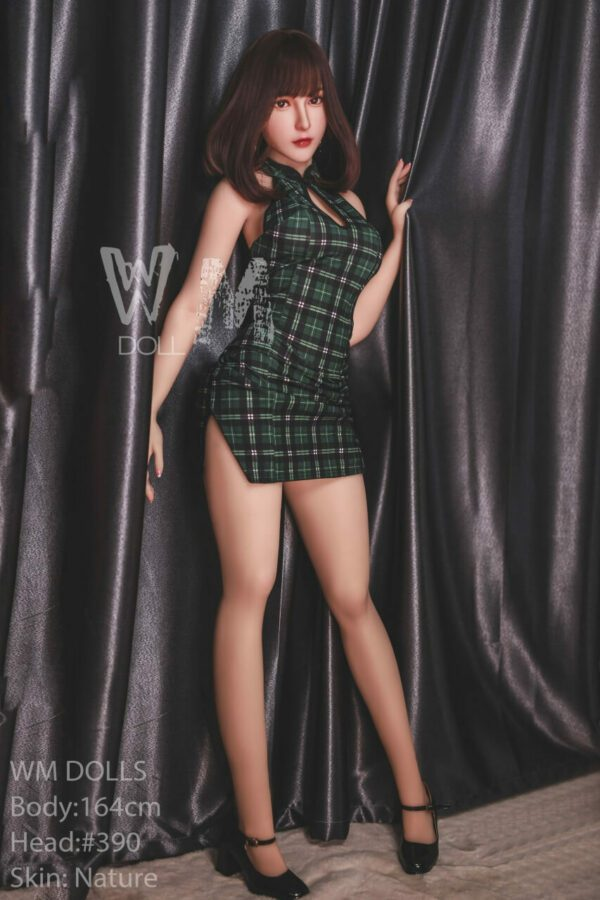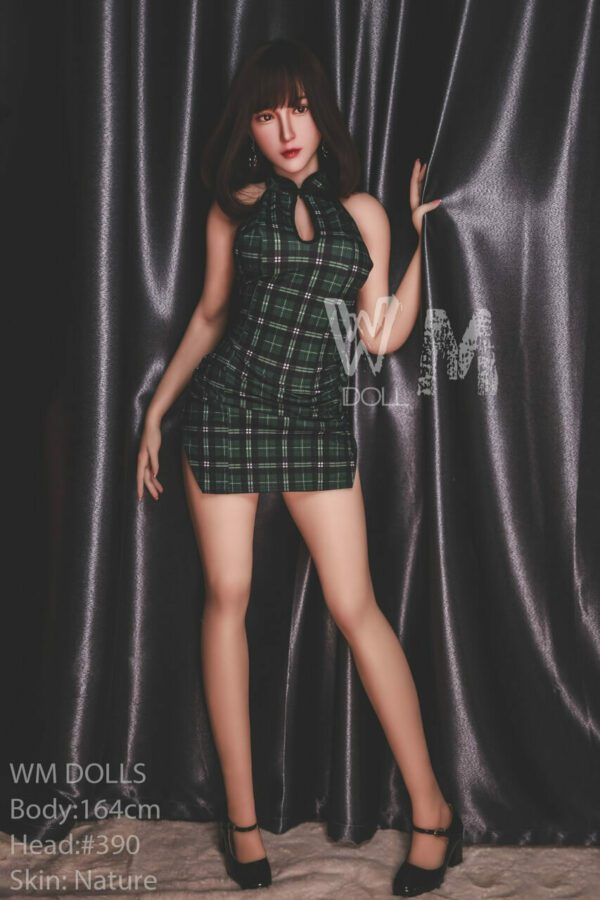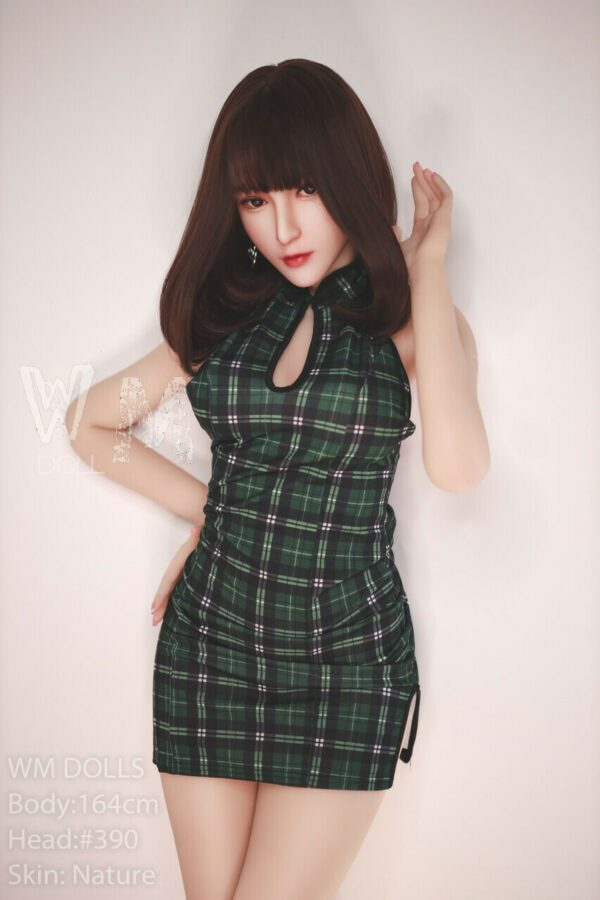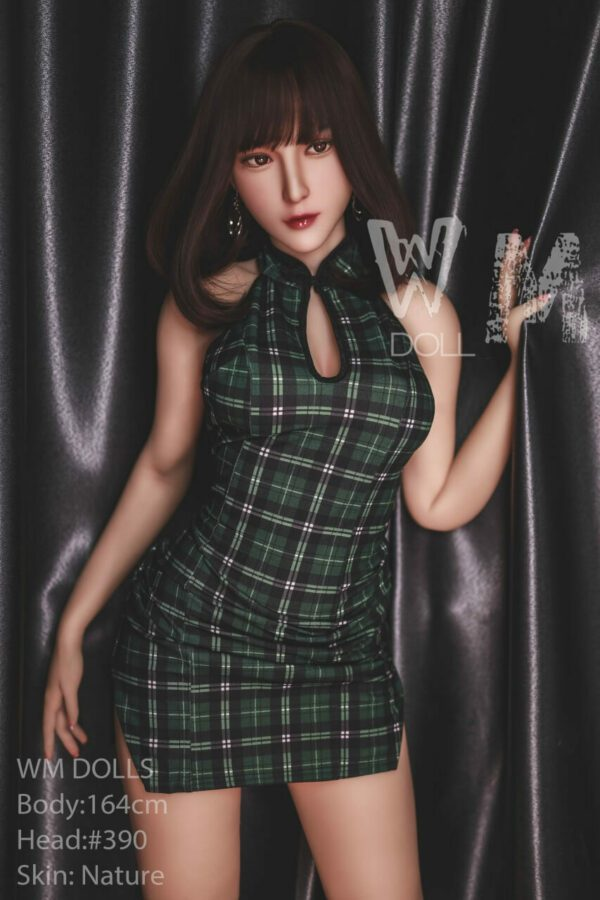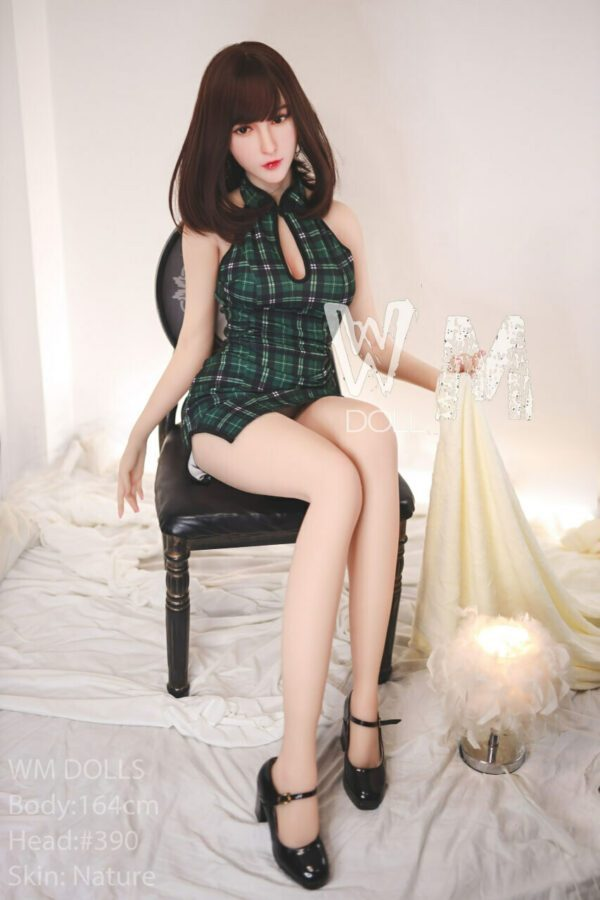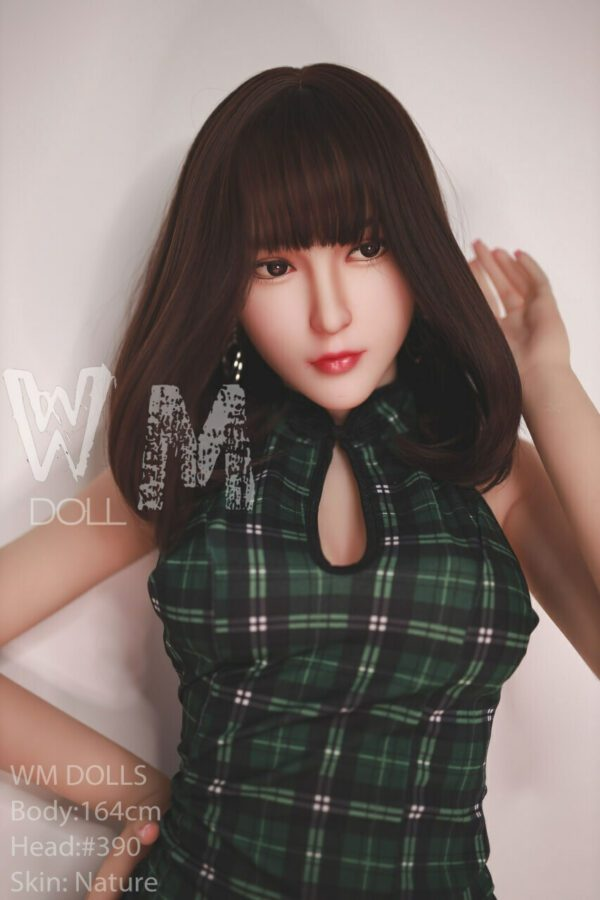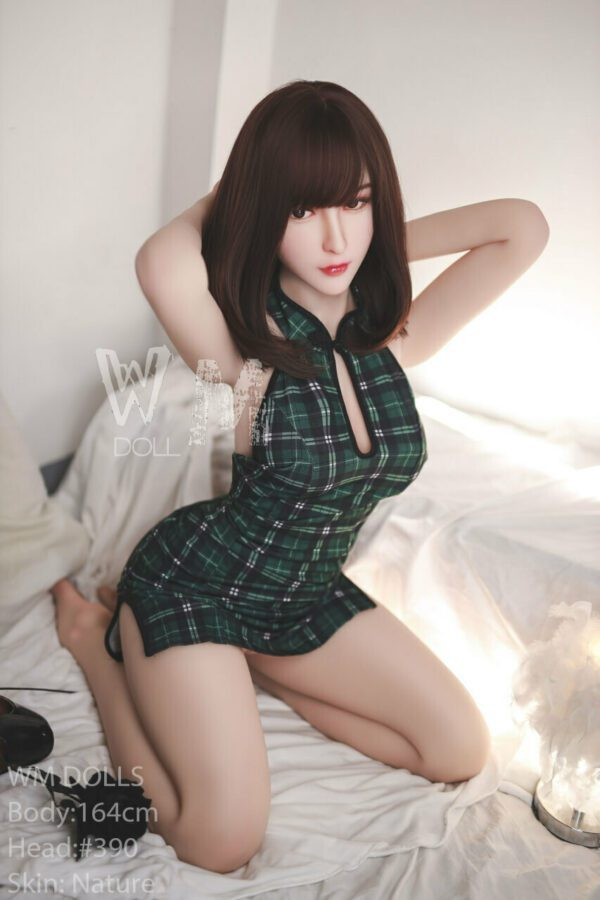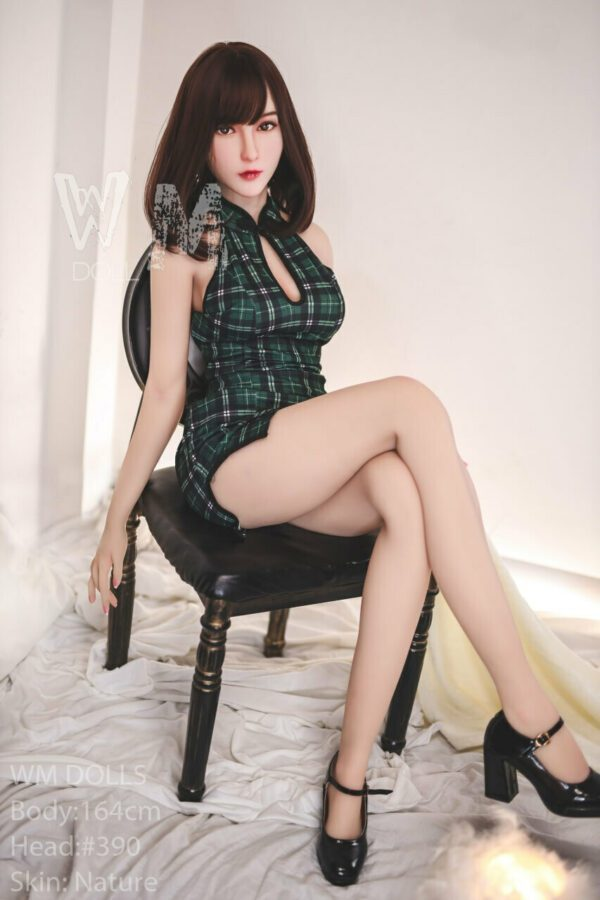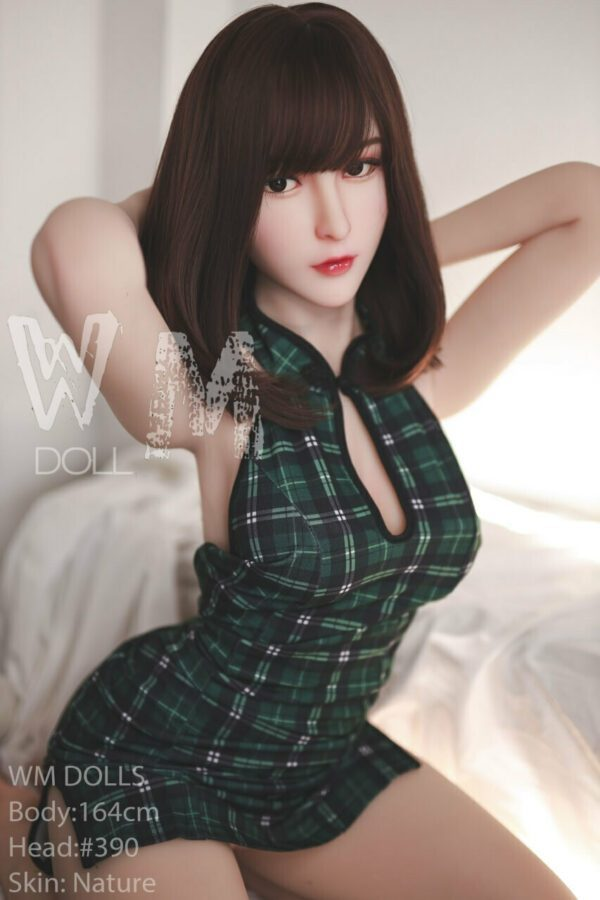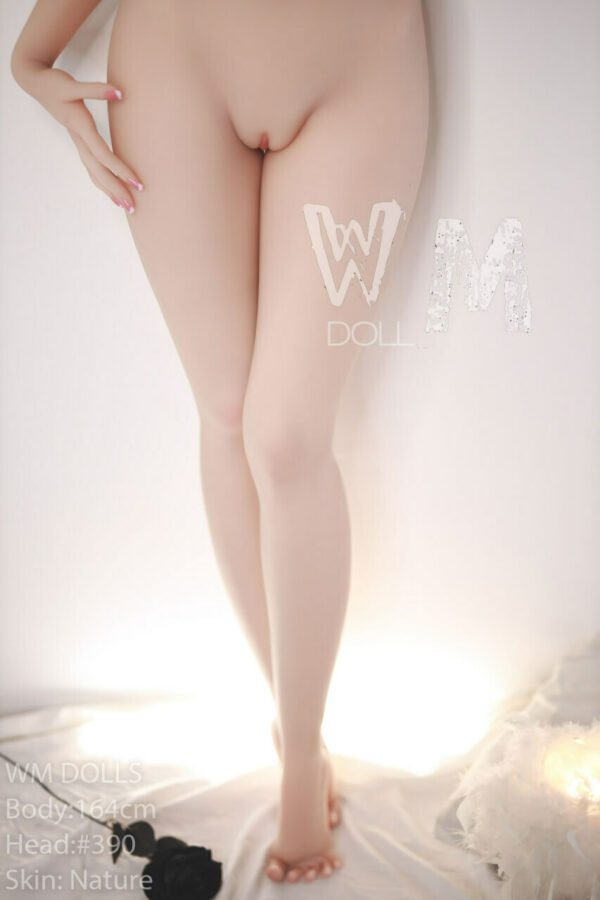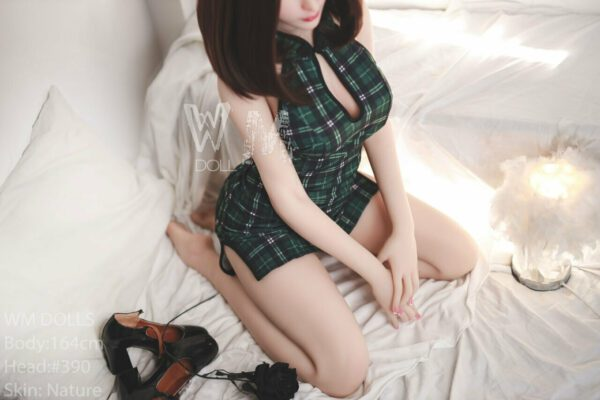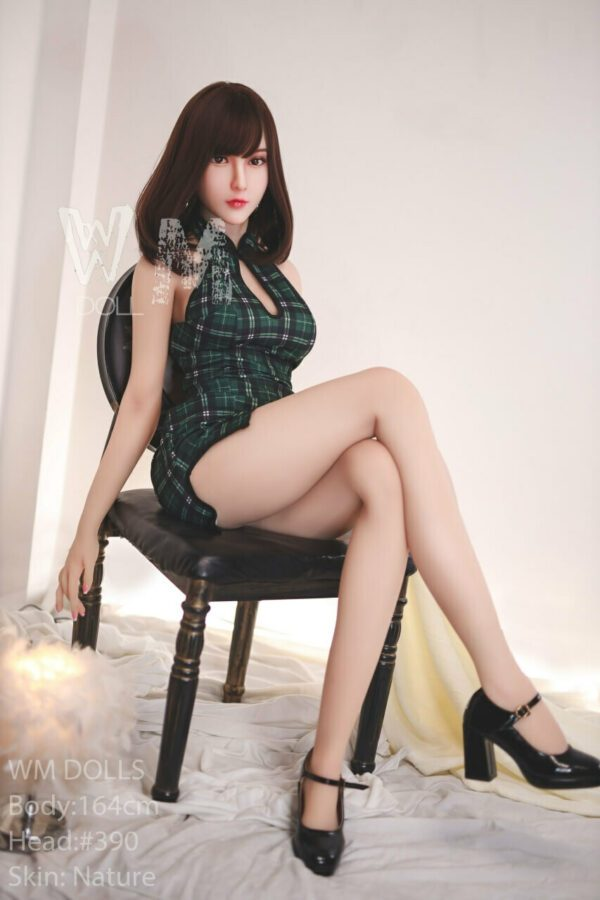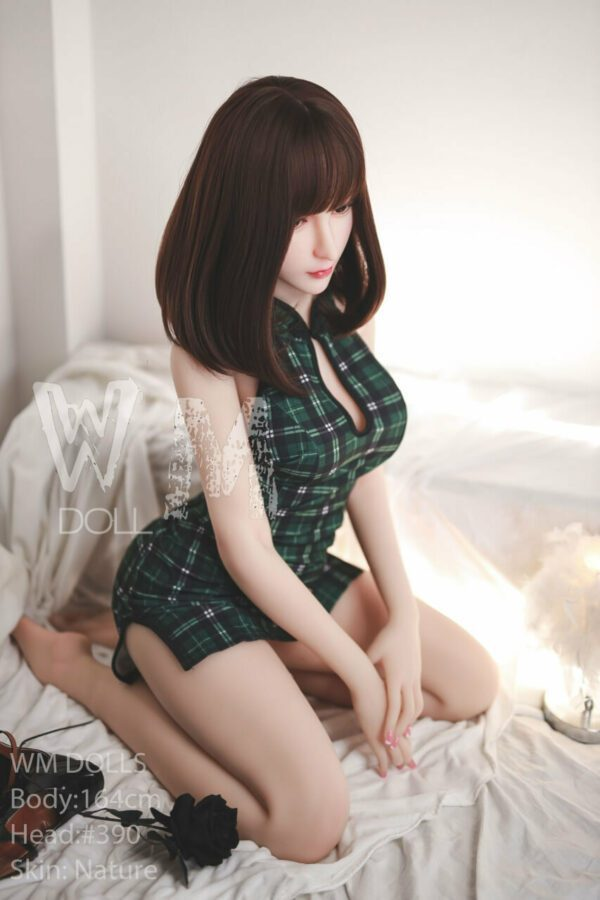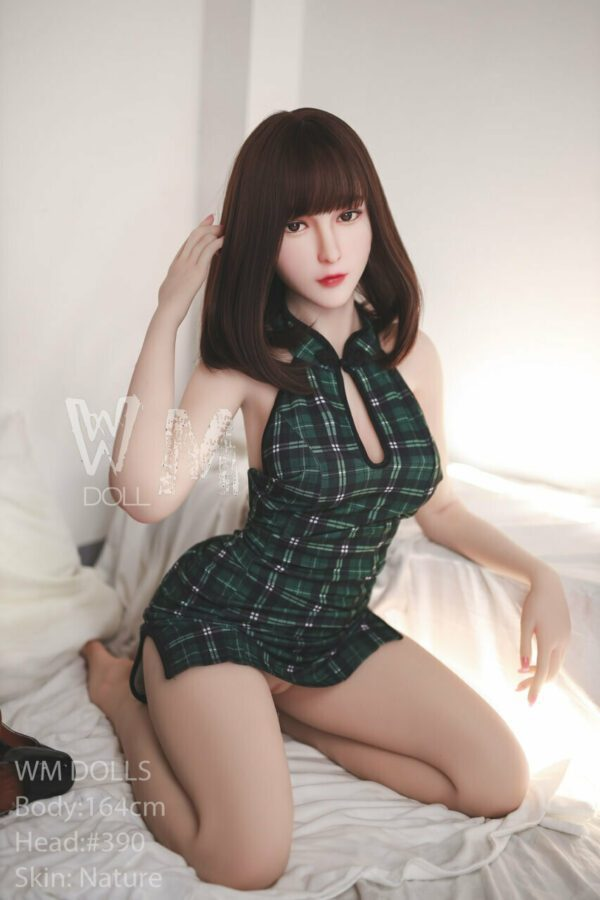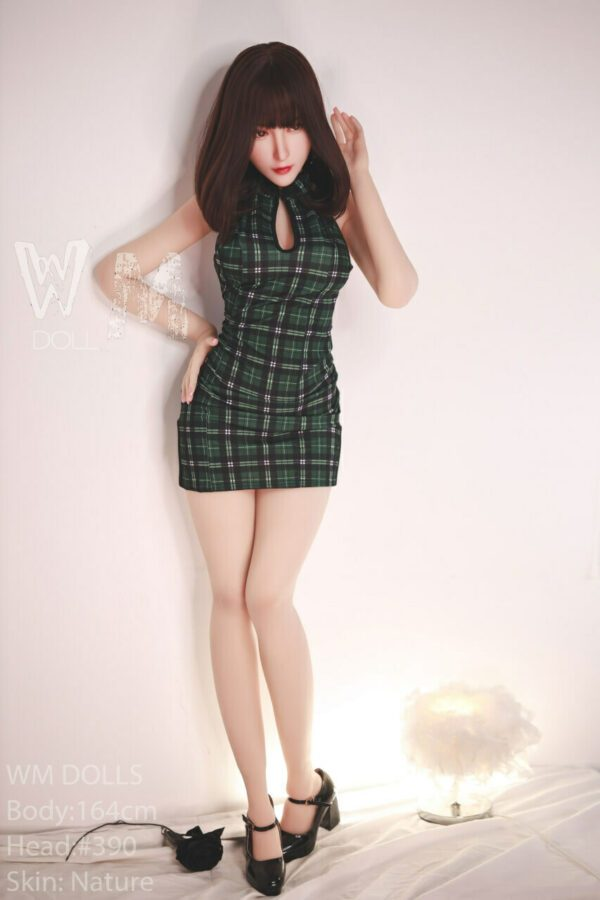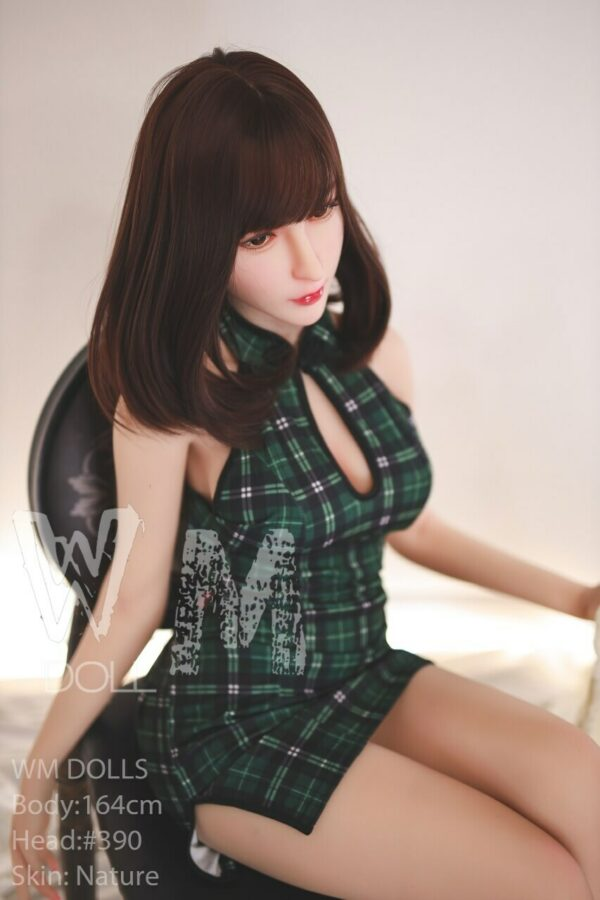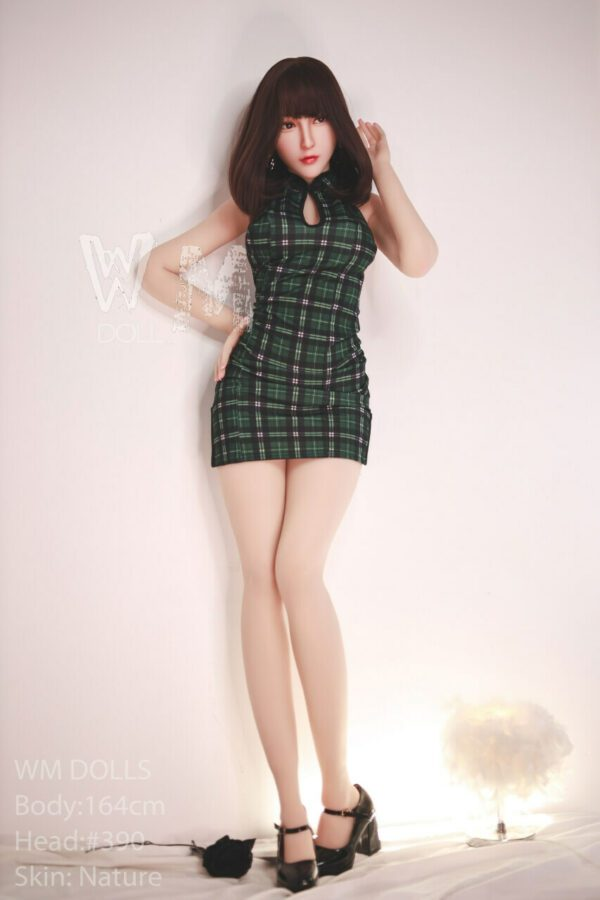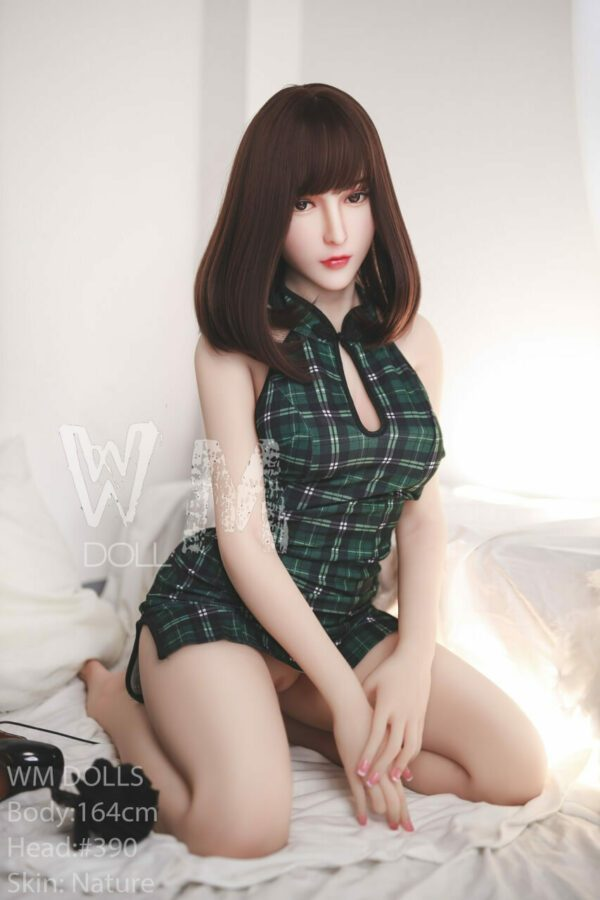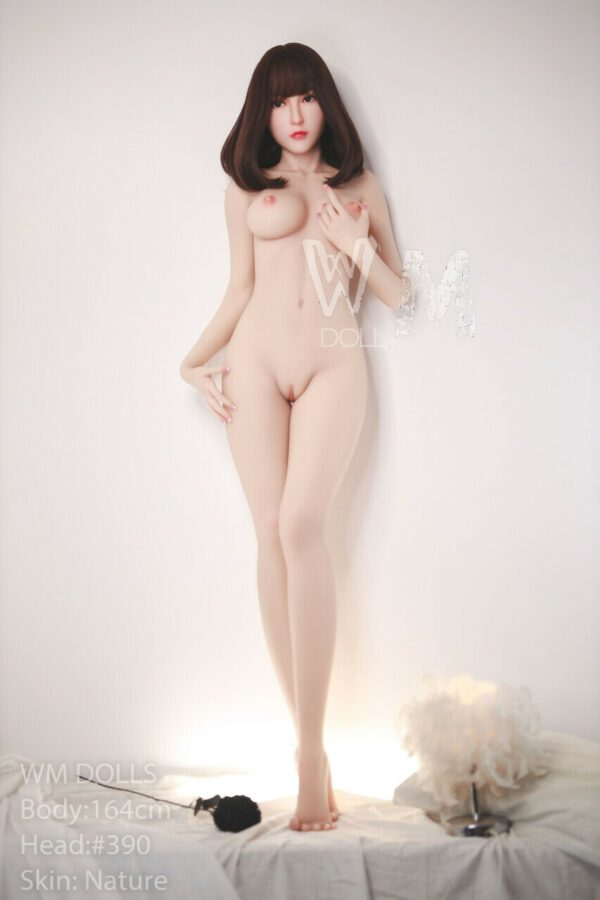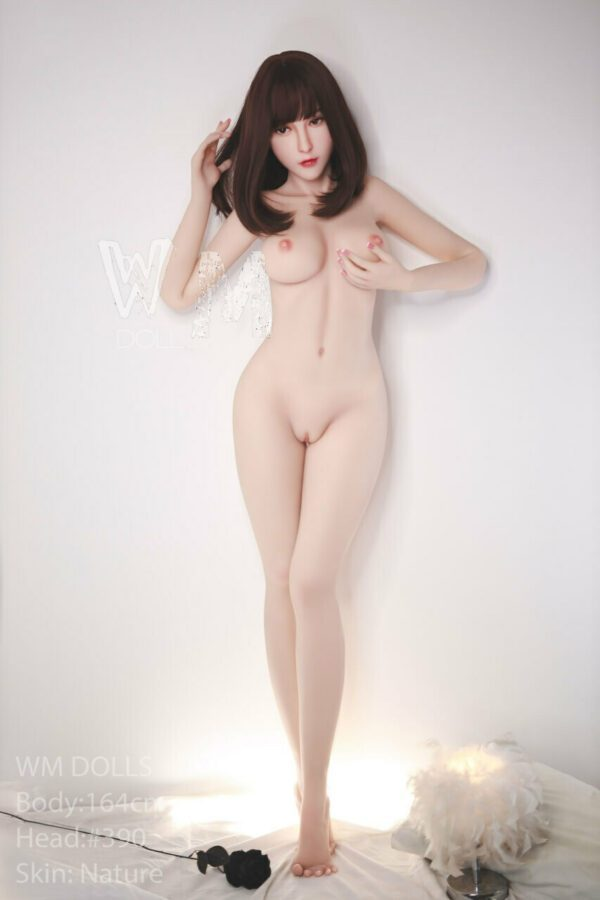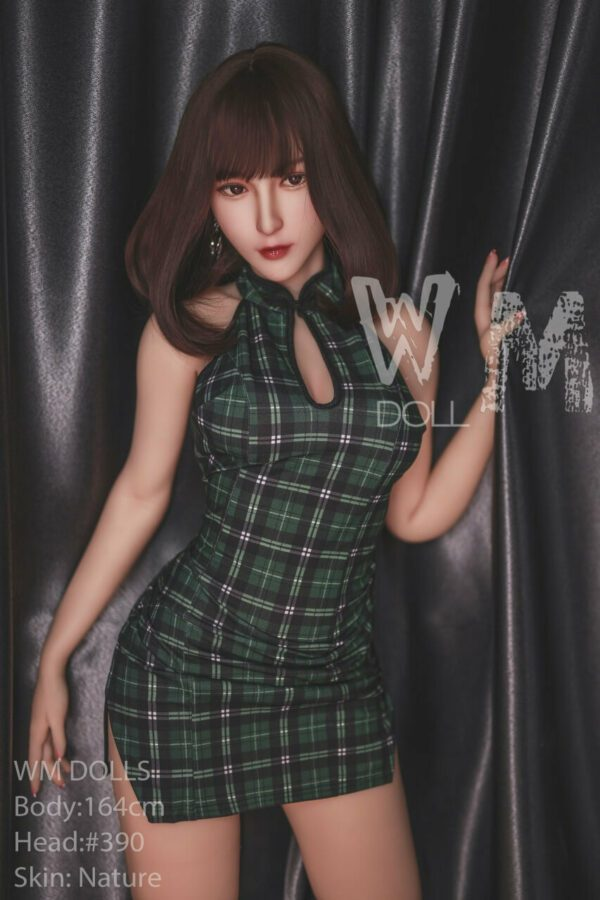# Inkeri – 5’5″ | 164cm Full Body Sex Doll

WM Doll
\$1,700

Inkeri – 5’5″ | 164cm Full Body Sex Doll made with high quality and safety standards by WM Doll, this genuine silicone and TPE sexy real sex doll is CE certified. With various features complementing each other, this realistic sex doll is sure to please the owner.

## Description

Height > 5ft 3.5 in – 5ft 5in (161cm – 165cm), Ethnicity > Asian, Hair Color > Brunette, Age > Eighteen & Nineteen, Material > Fully TPE, Breast Size > Medium, See All Sex Dolls, Body Style > Slender, BRANDS > WM Doll, Age > Young Adult

• \$
• \$
• \$
• \$
• \$
• \$
• \$
• \$
• \$
• \$
• \$
• \$
• \$
• \$
• \$
• \$
• \$
• \$
• \$
• \$
• \$
• \$
• \$
• \$
• \$
• \$
• \$
• \$
• \$
• \$
• \$
• \$
• \$
• \$
• \$
• \$
• \$
• \$
• \$
• \$
• \$
• \$
• \$
• \$
• \$
• \$
• \$
• \$
• \$
• \$
• \$
• \$
• \$
• \$
• \$
• \$
• \$
• \$
• \$
• \$
• \$
• \$
• \$
• \$
• \$
• \$
• \$
• \$
• \$
• \$
• \$
• \$
• \$
• \$
• \$
• \$
• \$
• \$
• \$
• \$
• \$
• \$
• \$
• \$
• \$
• \$
• \$
• \$
• \$
• \$
• \$
• \$
• \$
• \$
• \$
• \$
• \$
• \$
• \$
• \$
• \$
• \$
• \$
• \$
• \$
• \$
• \$
• \$
• \$
• \$
• \$
• \$
• \$
• \$
• \$
• \$
• \$
• \$
• \$
• \$
• \$
• \$
• \$
• \$
• \$
• \$
• \$
• \$
• \$
• \$
• \$
• \$
• \$
• \$
• \$
• \$
• \$

• \$
• \$
• \$
• \$
• \$
• \$
• \$

• \$

• \$
• \$
• \$
• \$
• \$
• \$
• \$
• \$
• \$
• \$
• \$
• \$
• \$
• \$
• \$
• \$
• \$
• \$
• \$
• \$
• \$

• \$
• \$
• \$
• \$
• \$
• \$
• \$
• \$
• \$
• \$
• \$
• \$
• \$
• \$
• \$
• \$
• \$
• \$
• \$
• \$

• \$
• \$
• \$
• \$
• \$
• \$
• \$

• \$
• 40 \$

• \$
• 100 \$

• \$
• \$
• \$
• \$
• \$

• \$
• \$
• \$
• \$
• \$

• \$
• \$
• \$

• \$
• 130 \$

• \$
• \$
• \$

• \$
• 40 \$

• \$
• \$
• \$
• \$

• \$
• \$
• \$
• \$
• \$

• \$
• \$

• \$
• \$
• \$
• \$
• \$
• \$
• \$
• \$
• \$
• \$

• \$
• \$
• \$
• \$
• \$
• \$

• \$
• 200 \$

• \$
• 100 \$

• \$
• 675 \$

• \$
• 390 \$

## Doll Measurements

Height: 164 cm | 5 ft 4.1 in
Weight: 33.9kg | 74.7 lbs.
Shoulder: 36 cm | 14.2 in
Full Bust: 82 cm | 32.3 in
Under Bust: 57 cm | 22.4 in
Cup Size: D
Waist: 53.5 cm | 21 in
Hips: 88 cm | 34.6 in
Foot Length: 21 cm | 8.3 in
Vaginal Depth: 18cm | 7 in
Anal Depth: 15 cm | 5.9 in
Oral Depth: 12 cm | 4.7 cm